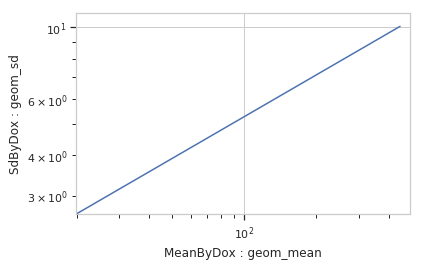# cytoflow.views.stats_2d¶

class cytoflow.views.stats_2d.Stats2DView[source]

Plot two statistics on a scatter plot. A point (X,Y) is drawn for every pair of elements with the same value of variable; the X value is from xstatistic and the Y value is from ystatistic.

xstatistic, ystatistic

The name of the statistics to plot. Must be a keys in the statistics attribute of the Experiment being plotted.

Type: (str, str)
x_error_statistic, y_error_statistic

The name of the statistics used to plot error bars. Must be keys in the statistics attribute of the Experiment being plotted.

Type: (str, str)
xscale, yscale

The scales applied to the data before plotting it.

Type: {‘linear’, ‘log’, ‘logicle’}
variable

The condition that varies when plotting this statistic: used for the x axis of line plots, the bar groups in bar plots, etc.

Type: str
subset

An expression that specifies the subset of the statistic to plot.

Type: str
xfacet, yfacet

Set to one of the conditions in the Experiment, and a new row or column of subplots will be added for every unique value of that condition.

Type: String
huefacet

Set to one of the conditions in the in the Experiment, and a new color will be added to the plot for every unique value of that condition.

Type: String
huescale

How should the color scale for huefacet be scaled?

Type: {‘linear’, ‘log’, ‘logicle’}

Examples

Make a little data set.

>>> import cytoflow as flow
>>> import_op = flow.ImportOp()
>>> import_op.tubes = [flow.Tube(file = "Plate01/RFP_Well_A3.fcs",
...                              conditions = {'Dox' : 10.0}),
...                    flow.Tube(file = "Plate01/CFP_Well_A4.fcs",
...                              conditions = {'Dox' : 1.0})]
>>> import_op.conditions = {'Dox' : 'float'}
>>> ex = import_op.apply()


Create two new statistics

>>> ch_op = flow.ChannelStatisticOp(name = 'MeanByDox',
...                     channel = 'Y2-A',
...                     function = flow.geom_mean,
...                     by = ['Dox'])
>>> ex2 = ch_op.apply(ex)
>>> ch_op_2 = flow.ChannelStatisticOp(name = 'SdByDox',
...                       channel = 'Y2-A',
...                       function = flow.geom_sd,
...                       by = ['Dox'])
>>> ex3 = ch_op_2.apply(ex2)


Plot the statistics

>>> flow.Stats2DView(variable = 'Dox',
...                  xstatistic = ('MeanByDox', 'geom_mean'),
...                  xscale = 'log',
...                  ystatistic = ('SdByDox', 'geom_sd'),
...                  yscale = 'log').plot(ex3)enum_plots(experiment)[source]

Returns an iterator over the possible plots that this View can produce. The values returned can be passed to plot().

plot(experiment, plot_name=None, **kwargs)[source]

Plot a chart of two statistics’ values as a common variable changes.

Parameters: experiment (Experiment) – The Experiment to plot using this view. title (str) – Set the plot title xlabel, ylabel (str) – Set the X and Y axis labels huelabel (str) – Set the label for the hue facet (in the legend) legend (bool) – Plot a legend for the color or hue facet? Defaults to True. sharex, sharey (bool) – If there are multiple subplots, should they share axes? Defaults to True. height (float) – The height of each row in inches. Default = 3.0 aspect (float) – The aspect ratio of each subplot. Default = 1.5 col_wrap (int) – If xfacet is set and yfacet is not set, you can “wrap” the subplots around so that they form a multi-row grid by setting col_wrap to the number of columns you want. sns_style ({“darkgrid”, “whitegrid”, “dark”, “white”, “ticks”}) – Which seaborn style to apply to the plot? Default is whitegrid. sns_context ({“paper”, “notebook”, “talk”, “poster”}) – Which seaborn context to use? Controls the scaling of plot elements such as tick labels and the legend. Default is talk. despine (Bool) – Remove the top and right axes from the plot? Default is True. xlim, ylim ((float, float)) – Set the range of the plot’s axis. color (a matplotlib color) – The color to plot with. Overridden if huefacet is not None linestyle ({‘solid’ | ‘dashed’, ‘dashdot’, ‘dotted’ | (offset, on-off-dash-seq) | ‘-‘ | ‘–’ | ‘-.’ | ‘:’ | ‘None’ | ‘ ‘ | ‘’}) marker (a matplotlib marker style) – See http://matplotlib.org/api/markers_api.html#module-matplotlib.markers markersize (int) – The marker size in points markerfacecolor (a matplotlib color) – The color to make the markers. Overridden (?) if huefacet is not None alpha (the alpha blending value, from 0.0 (transparent) to 1.0 (opaque))

Notes

Other kwargs are passed to matplotlib.pyplot.plot